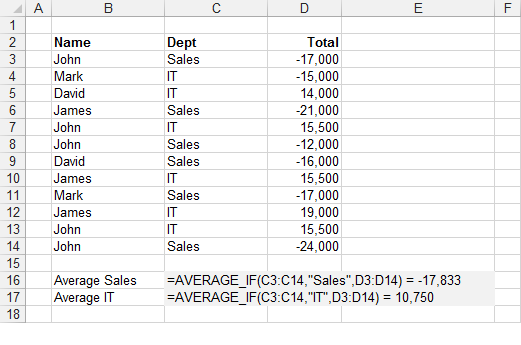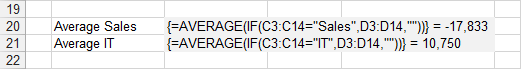# AVERAGEIFS Function

The AVERAGEIFS function returns the arithmetic mean of all the numbers in a range that satisfies multiple conditions.### Array Formula

To find out more about Array Formulas please refer to the Array Formulas section.
To find out more about the individual functions please refer to the AVERAGE function and IF function pages.
This example illustrates how to use an array formula to obtain the average value in a column based on a condition.The formula in row 16 returns the average in the "Sales" department.
The formula in row 17 returns the average in the "IT" department.
The formula [C3:C14="Sales"] returns an array of 1's and 0's indicating if the value in column "C" satisfies the condition.
Every number 1 in this array represents the value True in the IF statement and therefore has its corresponding value returned from column "D".
Every number 0 in this array represents the value False in the IF statement and therefore an empty string is returned in this case. The MAX function will ignore any string values.
The use of the empty string is to ensure that the formula works with both positive and negative numbers.
This array of numbers is then passed to the AVERAGE function to obtain the largest number.
Remember that you must press (Ctrl + Shift + Enter) to enter the formulas.
You can also use the SUMPRODUCT Function